# 算法概述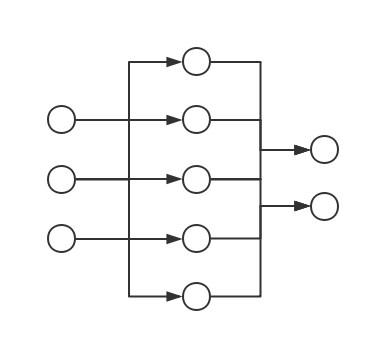• 记上图中的神经网络从左到右对应的 Layer 为$L_{1},L_{2},L_{3}$、记$L_{i}$中从上往下数的第 j 个神经元为$u_{ij}$
• $L_{i}$对应的：
• 神经元个数为$n_{i}$（从而$n_{1} = 3$$n_{2} = 5$$n_{3} = 2$
• 激活函数、偏置量分别为$\phi_{i}$$b^{\left( i \right)}$（注意$b^{\left( 3 \right)}$其实不会被用到）
• $L_i,L_{i+1}$之间的权值矩阵为$w^{(i)}$、神经元$u_{ij},u_{i+1,k}$之间的权值为$w_{jk}^{(i)}$，可知：
• $L_{i}$对应的输入、输出为$u^{\left( i \right)},v^{\left( i \right)}$
• 记模型的输入、输出集为$X$$Y$，样本数为 N，损失函数为$L$；一般我们会要求$L$是一个二元对称函数，亦即对于$L$的输入空间中的任意两个向量（矩阵）$p$$q$都有：

• $u^{\left( 1 \right)} = X$$v^{\left( 1 \right)} = \phi_{1}(u^{\left( 1 \right)})$，注意$u^{\left( 1 \right)}$$v^{\left( 1 \right)}$都是$N \times 3$维矩阵
• $u^{\left( 2 \right)} = v^{\left( 1 \right)} \times w^{\left( 1 \right)} + b^{\left( 1 \right)}$$v^{\left( 2 \right)} = \phi_{2}(u^{\left( 2 \right)})$，注意$w^{\left( 1 \right)}$$3 \times 5$的矩阵、所以$u^{\left( 2 \right)}$$v^{\left( 2 \right)}$都是$N \times 5$维矩阵
• $u^{\left( 3 \right)} = v^{\left( 2 \right)} \times w^{\left( 2 \right)} + b^{\left( 2 \right)}$$v^{\left( 3 \right)} = \phi_{3}(u^{\left( 3 \right)})$，注意$w^{\left( 2 \right)}$$5 \times 2$的矩阵、所以$u^{\left( 3 \right)}$$v^{\left( 3 \right)}$都是$N \times 2$维矩阵

# 激活函数

## 逻辑函数（Sigmoid）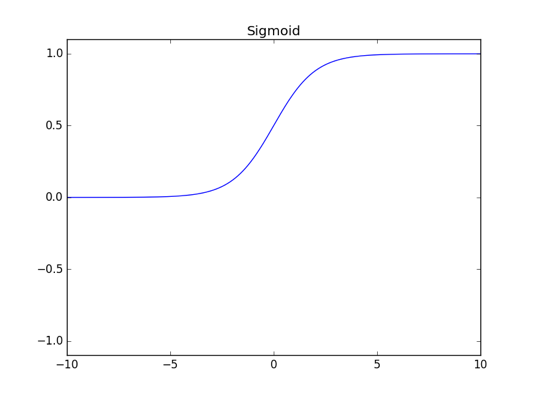## 正切函数（Tanh）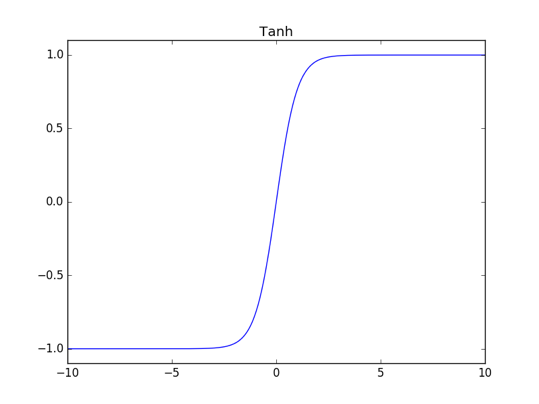## 线性整流函数（ReLU）

ReLU 的全称是 Rectified Linear Unit，定义式很简洁：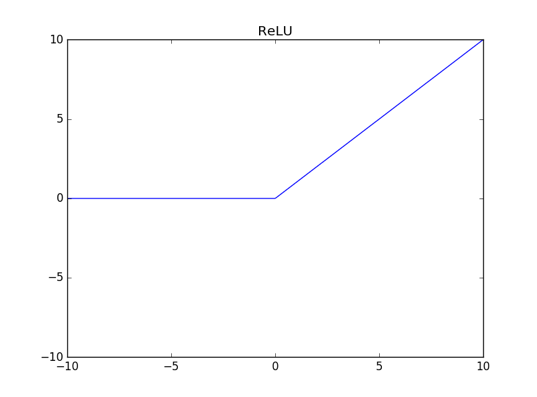## ELU 函数（Exponential Linear Unit）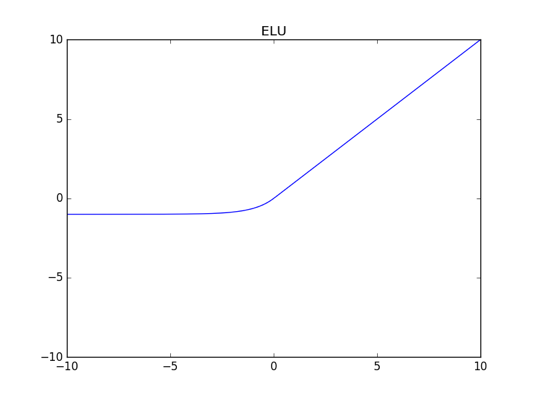## Softplus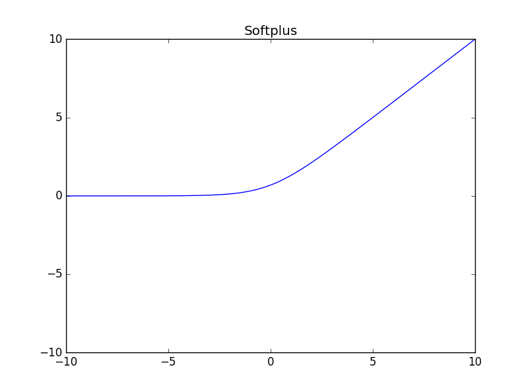## 恒同映射（Identify）

• $w$能把从激活函数得到的函数值线性映射到另一个维度的空间上
• $b$能在此基础上再进行一步平移的操作

• 激活函数全是中心对称的函数（比如常见的 tanh 函数）、亦即：
• 训练样本集为：
• 权值矩阵$w^{\left( 1 \right)}$$w^{\left( 2 \right)}$可变但偏置量恒为 0

# 损失函数（Cost Function）

• 假设样本为$(x,y)$
• 假设共有 K 类：$\{ c_{1},\ldots,c_{K}\}$
• 假设讨论的模型为$G$、其输出（向量）为$G(x)$

• 距离损失函数 该损失函数对应着最小平方误差准则（Minimum Squared Error，常简称为 MSE），它的直观意义是明确的：模型预测和真值的（欧氏）距离越大、损失就越大，反之就越小
• 交叉熵损失函数（要求$G(x)$每一位的取值都在$(0,1)$中） 其中交叉熵（Cross Entropy）是信息论中的一个概念、其本身是有一定内涵的，感兴趣的观众老爷可以参见维基百科来了解背后的那一套数学理论。囿于篇幅、我们无法展开叙述这一部分，但是从交叉熵的名字就可以看出、它和决策树里面提到过的熵有千丝万缕的关系；考虑到熵是定义在概率分布上的、所以进一步要求是一个概率向量（亦即进一步要求$\sum_{k=1}^Kv_k=1$）是一个非常合理的做法
• log-likelihood 损失函数（要求$G(x)$是一个概率向量、以及不妨假设$y\in c_k$换句话说，log-likelihood 即为模型预测的、真值 y 对应的类（$c_k$）的概率的负对数。需要指出的是、log-likelihood 这种原始的定义方式在神经网络里面不尽合理，我们会在下一节进行相关的讨论